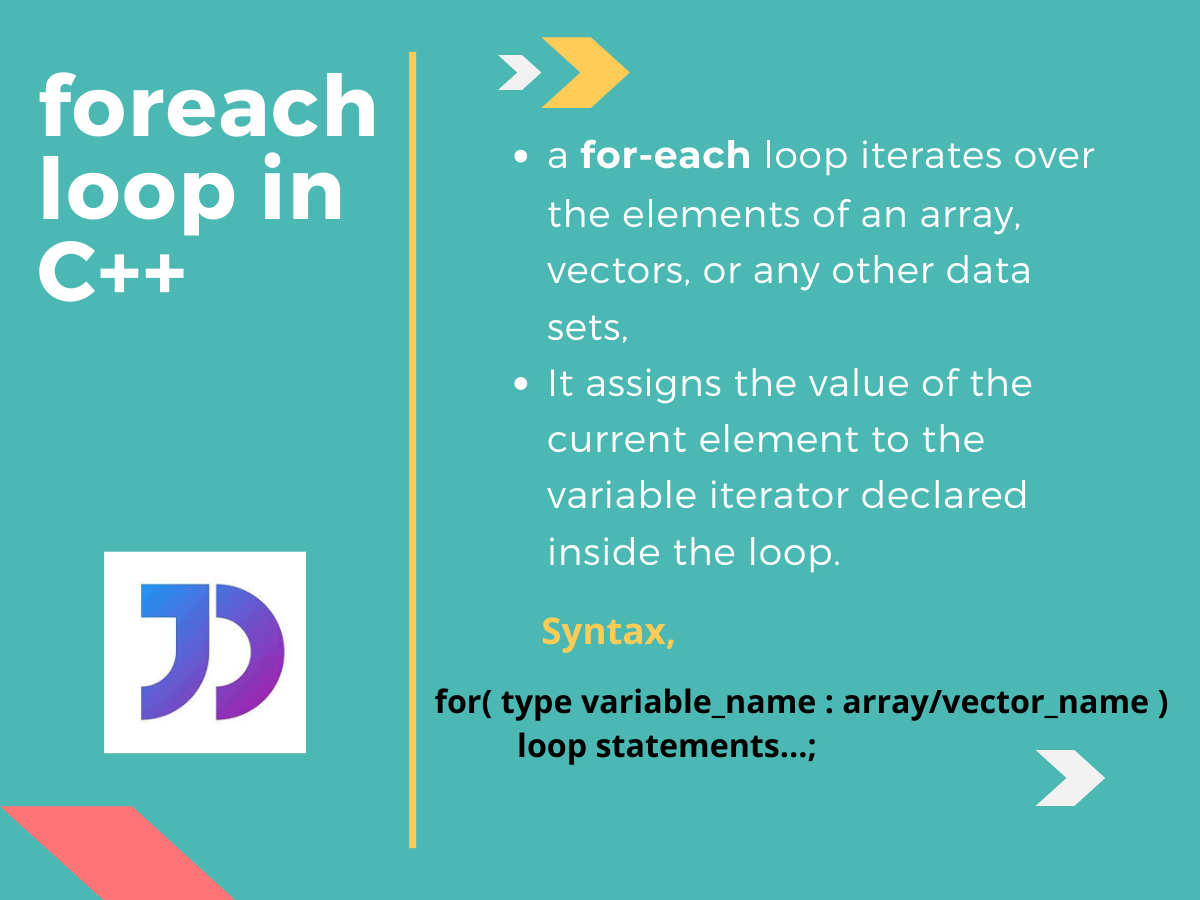# The foreach loop in C++

Published on August 3, 2022By Sneh
Developer and author at DigitalOcean.While we believe that this content benefits our community, we have not yet thoroughly reviewed it. If you have any suggestions for improvements, please let us know by clicking the “report an issue“ button at the bottom of the tutorial.

## Introduction

The foreach loop in C++ or more specifically, range-based for loop was introduced with the C++11. This type of for loop structure eases the traversal over an iterable data set. It does this by eliminating the initialization process and traversing over each and every element rather than an iterator. So let us dig into the respective foreach loop structure.

## Working of the foreach loop in C++

So basically a for-each loop iterates over the elements of arrays, vectors, or any other data sets. It assigns the value of the current element to the variable iterator declared inside the loop. Let us take a closer look at the syntax:

``````for(type variable_name : array/vector_name)
{
loop statements
...
}
``````

As we can see:

• During the loop initialization, the elemental variable declaration is the part where we need to declare the variable which will iterate over the array or vector. Here, the `type` is the data type of the variable_name
• array/vector name is the name of the respective data set over which the loop will iterate,
• loop statements are the different operations which the user can choose to perform over the corresponding elements with the use of the iterating variable.

Note: It is suggested to keep the data type of the variable the same as that of the array or vector. If the data type is not the same, then the elements are going to be type-casted and then stored into the variable.

## Examples of foreach loop

### 1. Example of foreach loop for Arrays in C++

The code given below illustrates the use of the for-each loop in C++,

``````#include<iostream>
using namespace std;
int main()
{
int arr[]={1,2,3,4,5};   //array initialization
cout<<"The elements are: ";
for(int i : arr)
{
cout<<i<<" ";
}
return 0;
}
``````

Output:

``````The elements are: 1 2 3 4 5
``````

Let’s break down the code and look at it line-by-line:

• An array `arr[]` is initialized with some values {1 , 2 , 3 , 4 , 5}
• Inside the loop structure, ‘i’ is the variable that stores the value of the current array element
• `arr` is the array name which also serves as the base address of the respective array
• As we can see, printing ‘i’ for each iteration gives us the corresponding array elements in contrast to the array indices in case of normal for loop

Please note: While declaring the variable ‘i’ we could also use the `auto` datatype instead of `int`. This ensures that the type of the variable is deduced from the array type, and no data type conflicts occur.

For example:

``````#include<iostream>
using namespace std;
int main()
{
int array[]={1,4,7,4,8,4};
cout<<"The elements are: ";
for(auto var : array)
{
cout<<var<<" ";
}
return 0;
}
``````

Output:

### 2. Example of foreach loop for Vectors in C++

The following code illustrates the use of the for-each loop for iterating over a `vector`.

``````#include<iostream>
#include<vector>
using namespace std;
int main()
{
vector<int> vec={11,22,33,44,55,66};
cout<<"The elements are: ";
for(auto var : vec)
{
cout<<var<<" ";
}
return 0;
}
``````

Output:

The for-each loop for vector works in the same way as it does for an array. Furthermore, the only differences are the vector declaration, initialization and the different operations that can be performed over it.

### 1. Advantages of foreach loop

• It eliminates the possibility of errors and makes the code more readable.
• Easy to implement
• Does not require pre-initialization of the iterator

### 2. Disadvantages of foreach loop

• Cannot directly access the corresponding element indices
• Cannot traverse the elements in reverse order
• It doesn’t allow the user to skip any element as it traverses over each one of them

## Conclusion

The foreach loop in C++ has its own pros and cons. The code is easy to read but it restricts some of the actions that the normal for loop offers. Hence, it completely depends on the user what he/she wants the loop to perform and choose accordingly.